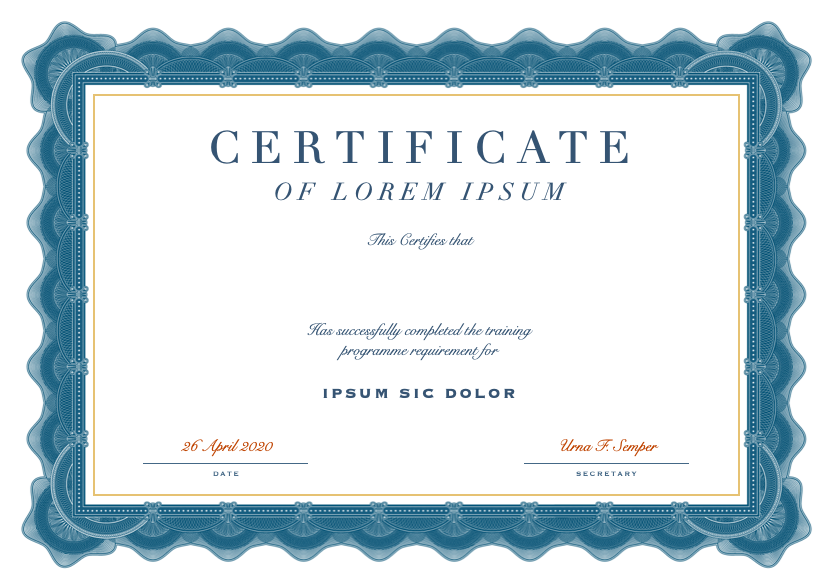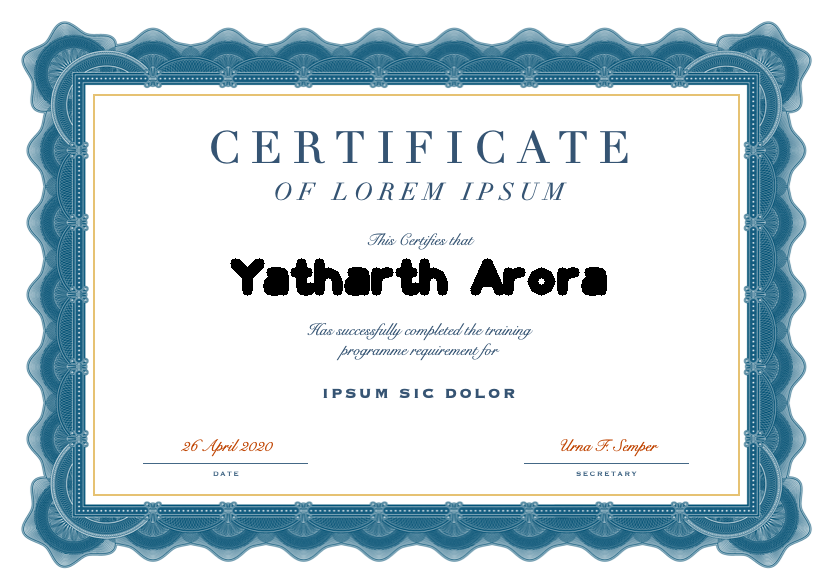Open In App

# Automated Certificate generator using Opencv in Python

Prerequisites: Introduction to OpenCV

OpenCV is the huge open-source library for computer vision, machine learning, and image processing and now it plays a major role in real-time operation which is very important in today’s systems. By using it, one can process images and videos to identify objects, faces, or even the handwriting of a human.

Any event usually involves a lot of participants and generating handwritten certificates for each one of them and sending them digitally is a really tedious task. Automating this job can easily save tons of time and manual work and thus also reducing the error rate. This Python script generates certificates with the persons name, reading from an excel file after loading a template certificate in the script.

Below is the implementation.

Certificate Template:`#import the necessary libraries`` ` `import` `cv2 as cv``import` `openpyxl``  ` `     ` `# template1.png is the template``# certificate``template_path ``=` `'template12.png'`` ` `# Excel file containing names of ``# the participants``details_path ``=` `'gsocOrgsList.xlsx'`` ` `# Output Paths``output_path ``=` `'/home/nikhil/Desktop/gfg'``  ` `# Setting the font size and font``# colour``font_size ``=` `3``font_color ``=` `(``0``,``0``,``0``)``  ` `# Coordinates on the certificate where``# will be printing the name (set``# according to your own template)``coordinate_y_adjustment ``=` `15``coordinate_x_adjustment ``=` `7``  ` `# loading the details.xlsx workbook ``# and grabbing the active sheet``obj ``=` `openpyxl.load_workbook(details_path)``sheet ``=` `obj.active``  ` `# printing for the first 10 names in the``# excel sheet``for` `i ``in` `range``(``1``,``11``):``      ` `    ``# grabs the row=i and column=1 cell ``    ``# that contains the name value of that``    ``# cell is stored in the variable certi_name``    ``get_name ``=` `sheet.cell(row ``=` `i ,column ``=` `1``)``    ``certi_name ``=` `get_name.value``                              ` `    ``# read the certificate template``    ``img ``=` `cv.imread(template_path)``                                ` `    ``# choose the font from opencv``    ``font ``=` `cv.FONT_HERSHEY_PLAIN              ``  ` `    ``# get the size of the name to be``    ``# printed``    ``text_size ``=` `cv.getTextSize(certi_name, font, font_size, ``10``)[``0``]     ``  ` `    ``# get the (x,y) coordinates where the``    ``# name is to written on the template``    ``# The function cv.putText accepts only``    ``# integer arguments so convert it into 'int'.``    ``text_x ``=` `(img.shape[``1``] ``-` `text_size[``0``]) ``/` `2` `+` `coordinate_x_adjustment ``    ``text_y ``=` `(img.shape[``0``] ``+` `text_size[``1``]) ``/` `2` `-` `coordinate_y_adjustment``    ``text_x ``=` `int``(text_x)``    ``text_y ``=` `int``(text_y)``    ``cv.putText(img, certi_name,``              ``(text_x ,text_y ), ``              ``font,``              ``font_size,``              ``font_color, ``10``)``  ` `    ``# Output path along with the name of the``    ``# certificate generated``    ``certi_path ``=` `output_path ``+` `'/certi'` `+` `'.png'``      ` `    ``# Save the certificate                      ``    ``cv.imwrite(certi_path,img)`

Output: